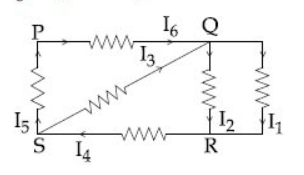# In the given circuit diagram, the currents, I1 = -0.3 A, I4 = 0.8 A and I5 = 0.4 A, areflowing as shown. The currents I2 , I3 and I6 ,respectively, are :Kirchoff's first law -- whereinApply Kcl at Q

I6+I3 = I1+ I2   .........(1)

I1 = 0.3A

I4 = 0.8A

I5 = 0.4A

KCL at 5

I4= I5 +I3

I3 = I4 - I5

= 0.8 +0.4

=0.4A

KCL at R

I1+I2 = I4

I2 = I4 - I1

= 0.8 - (-0.3)

=1.1A

From equation (1)

I6 + I3 = I1+ I2

I6 = I1 + I2 - I3

= -0.3+1.1 -0.4

=0.4A

I2 = 1.1A

I3 = 0.4A

I6 = 0.4A

## Most Viewed Questions

### Preparation Products

##### Knockout BITSAT 2022

An exhaustive program for the complete preparation of Physics Chemistry Mathematics English & Logical Reasoning of BITSAT exam, Adaptive Time Table, Chapterwise Questions, Concepts Flashcards for Quick and Effective Revision, Unlimited Chapter wise Subject wise and Full mock test for enhancing Speed & Accuracy,.

₹ 2999/- ₹ 1999/-
##### Engineering Prep Combo

An exhaustive e-learning program to boost your preparation for the leading engineering entrance exams like:-, BITSAT, VITEEE, MET, SRMJEEE, AEEE, MHT CET, AP EAPCET, TS EAMCET, Unlimited Mock Test for each exam..

₹ 9999/- ₹ 2999/-